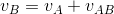## The Postulates of Special Relativity (3)

In the proof above, the Galilean velocity addition rule is utilized:. This rule applies to all relative motions. As it is Galileo who first brought up the idea of relative motion, this rule is named after him, to distinguish it from the velocity combination rule under special relativity.

The Galilean velocity addition rule is mostly used in mathematics as a meeting problem.

Let’s say we are separated by 10 km. If you walk 5 km an hour, and I walk 5 km an hour, then we can meet in 1 hour if we start at the same time. If you walk 6 km an hour, and I walk 4 km an hour, we still will meet in 1 hour. Why? Because the time required is determined by the distance separating us and our relative speed. In both situations, our relative speed is 10 km an hour.

When calculating this relative speed, what we used is the Galilean velocity addition rule.

In our proof of the principle of relativity, we used the Galilean velocity addition rule, which applies to all relative motions. Based on the Galilean concept that all motions are relative, we can see a hidden condition behind the principle of relativity: all objects follow the rule of relative motion.

For clarity, we can describe the first postulate like this:

• All reference frames of uniform motion are equivalent. Any physical law behaves the same in all of them. Or in another word, the mathematical form of a physical law is unique.
• All physical objects follow the rule of relative motion.